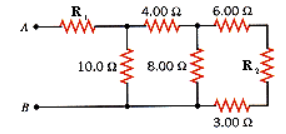# Problem: Find the equivalent resistance between points A and B in the drawing (R1 = 4.00, R2 = 8.00).

🤓 Based on our data, we think this question is relevant for Professor McElgin's class at LSU.

###### FREE Expert Solution

The equivalent resistance of series resistors,

$\overline{)\begin{array}{rcl}{\mathbf{R}}_{\mathbf{eq}}& {\mathbf{=}}& {\mathbf{R}}_{\mathbf{1}}\mathbf{+}{\mathbf{R}}_{\mathbf{2}}\mathbf{+}{\mathbf{R}}_{\mathbf{3}}\mathbf{.}\mathbf{.}\mathbf{.}\end{array}}$

The equivalent resistance of parallel resistors,

$\overline{)\begin{array}{rcl}\frac{\mathbf{1}}{{\mathbf{R}}_{\mathbf{eq}}}& {\mathbf{=}}& \frac{\mathbf{1}}{{\mathbf{R}}_{\mathbf{1}}}\mathbf{+}\frac{\mathbf{1}}{{\mathbf{R}}_{\mathbf{2}}}\mathbf{+}\frac{\mathbf{1}}{{\mathbf{R}}_{\mathbf{3}}}\mathbf{.}\mathbf{.}\mathbf{.}\end{array}}$

R3, RR2, and R6  are in series.

$\begin{array}{rcl}{\mathbf{R}}_{\mathbf{eq}}& \mathbf{=}& {\mathbf{R}}_{\mathbf{1}}\mathbf{+}{\mathbf{R}}_{\mathbf{2}}\mathbf{+}{\mathbf{R}}_{\mathbf{3}}\\ & \mathbf{=}& \mathbf{3}\mathbf{+}\mathbf{8}\mathbf{+}\mathbf{6}\end{array}$

R3,R2,6 = 17 Ω

R3,R2,6 and R8 are in parallel,###### Problem Details

Find the equivalent resistance between points A and B in the drawing (R1 = 4.00, R2 = 8.00).